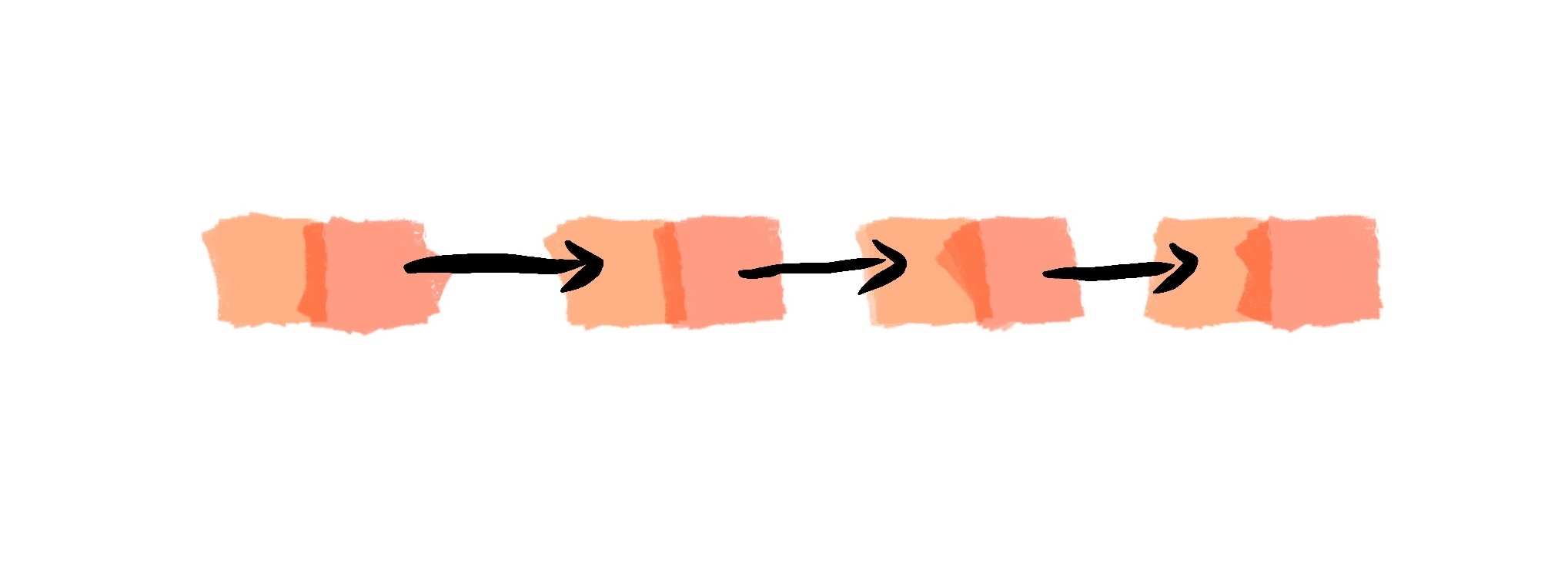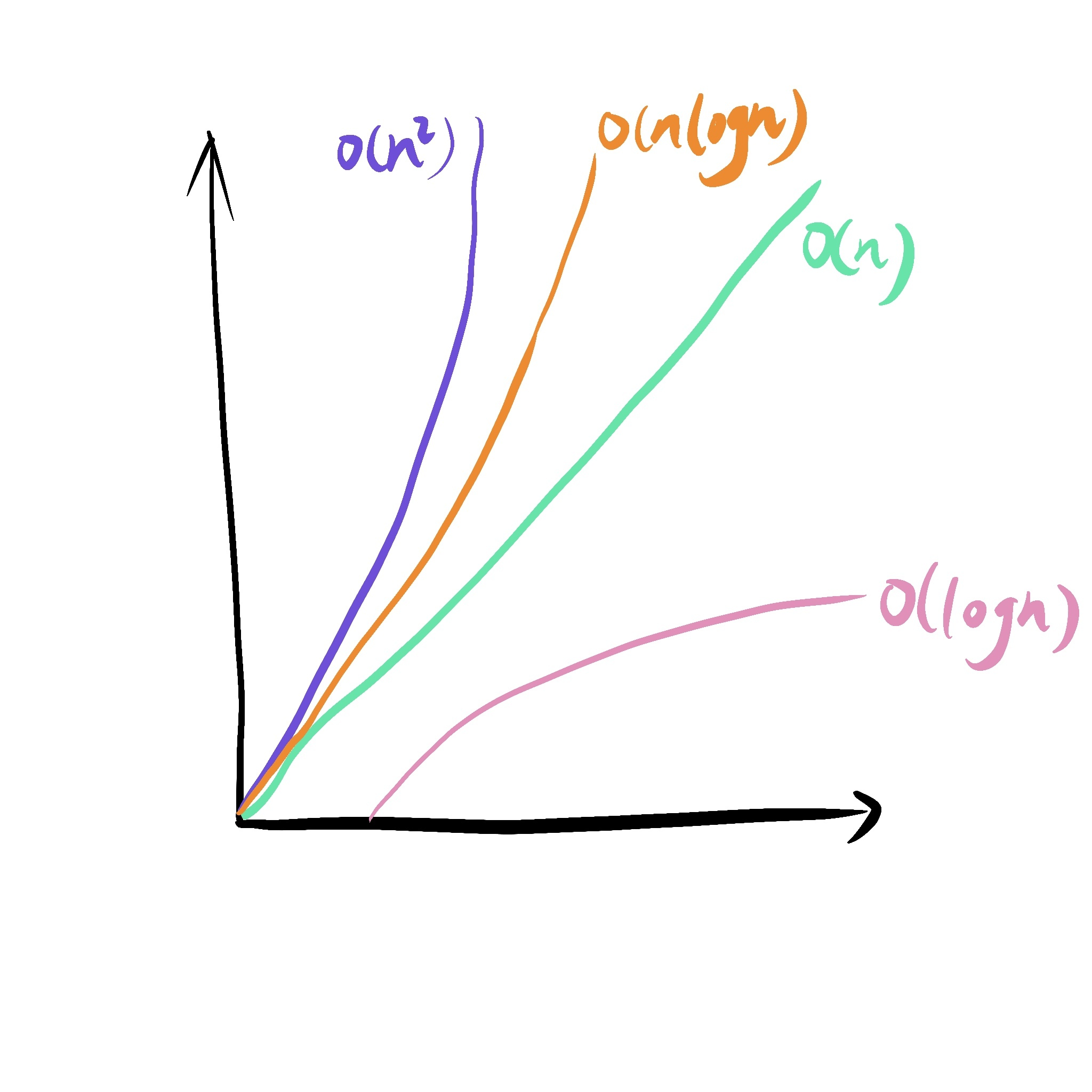面试指北：算法与数据结构（三）数组与链表3.141516数组与链表对比

时间复杂度

1. 只关注循环执行次数最多的代码，忽略其常量、低阶、系数;

2. 加法法则：一段代码的总复杂度，等于量级最大的那段代码的复杂度；

3. 乘法法则：嵌套代码的复杂度等于内外代码复杂度的乘积。

complexity(int n) {    int[] array = new int[n];
// 1: O(1) int a = array; int b = array;
// 2: O(n) for (int i = 0; i < n; i++) { print(array[i]); print(array[i]); }
// 3: o(n^2) for (int i = 0; i < n; i++) { for (int j = 0; j < n; j++) { print(array[i] * array[j]); } }}总结评论